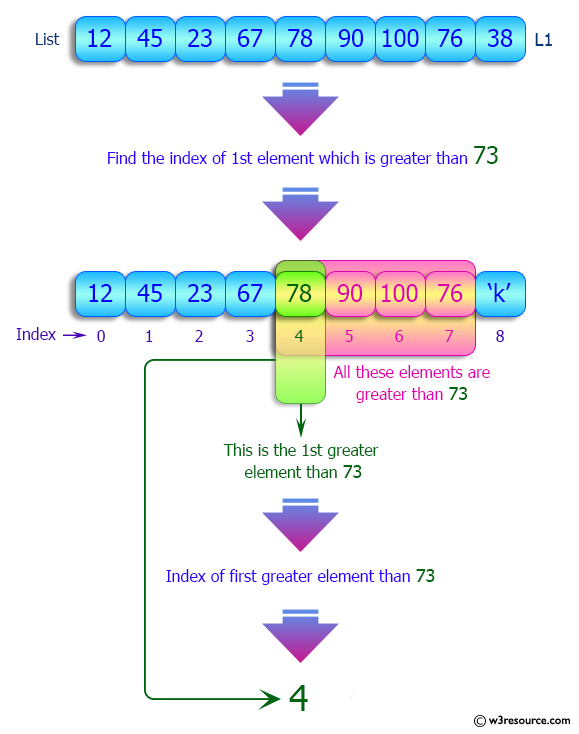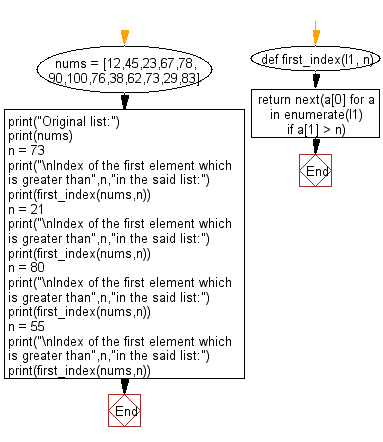﻿ Python: Index of the first element which is greater than a specified element - w3resource# Python: Index of the first element which is greater than a specified element

## Python List: Exercise - 163 with Solution

Write a Python program to get the index of the first element which is greater than a specified element.

Sample Solution:

Python Code:

``````def first_index(l1, n):
return next(a for a in enumerate(l1) if a > n)

nums = [12,45,23,67,78,90,100,76,38,62,73,29,83]
print("Original list:")
print(nums)
n = 73
print("\nIndex of the first element which is greater than",n,"in the said list:")
print(first_index(nums,n))
n = 21
print("\nIndex of the first element which is greater than",n,"in the said list:")
print(first_index(nums,n))
n = 80
print("\nIndex of the first element which is greater than",n,"in the said list:")
print(first_index(nums,n))
n = 55
print("\nIndex of the first element which is greater than",n,"in the said list:")
print(first_index(nums,n))
```
```

Sample Output:

```Original list:
[12, 45, 23, 67, 78, 90, 100, 76, 38, 62, 73, 29, 83]

Index of the first element which is greater than 73 in the said list:
4

Index of the first element which is greater than 21 in the said list:
1

Index of the first element which is greater than 80 in the said list:
5

Index of the first element which is greater than 55 in the said list:
3
```

Pictorial Presentation:Flowchart:## Visualize Python code execution:

The following tool visualize what the computer is doing step-by-step as it executes the said program:

Python Code Editor:

Have another way to solve this solution? Contribute your code (and comments) through Disqus.

What is the difficulty level of this exercise?

Test your Python skills with w3resource's quiz

﻿

## Python: Tips of the Day

Floor Division:

When we speak of division we normally mean (/) float division operator, this will give a precise result in float format with decimals.

For a rounded integer result there is (//) floor division operator in Python. Floor division will only give integer results that are round numbers.

```print(1000 // 300)
print(1000 / 300)```

Output:

```3
3.3333333333333335```